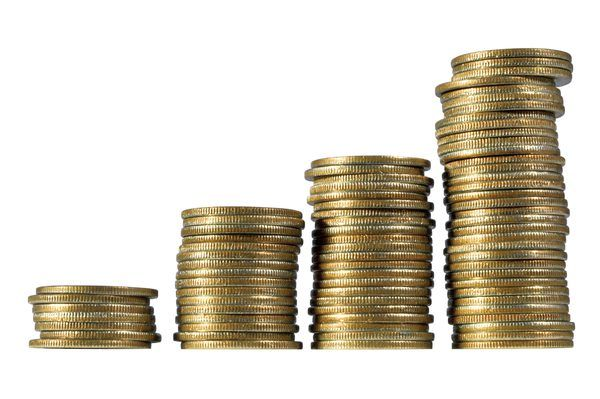Costs are a vital ingredient to evaluate fusion but, unfortunately, are very difficult to accurately evaluate at the present time. Fusion advocates suggest the electricity price from fusion will be competitive with fission. It is more likely that the actual cost of electricity from fusion would be several times that of fission.

### Estimates based on ITER construction costs

The construction cost of ITER is not completely transparent because of the in-kind contributions which are costed in different ways by the different countries involved. Estimates exist in the range \$22-65 billion. Michel Claessens estimates the total ITER cost based on the known EU contribution, which is 45.6% of the total, to be €41 billion (~\$44 billion). The US Dept of Energy cost estimate is the high end, \$65 billion.

Notwithstanding the complexity of using these figures to predict the cost of commercial fusion power, a number of people have made such estimates. Reinders in his book, The Fairy Tale of Nuclear Fusion, suggests fusion would be \$22000/KWh based on ITER costs, about 4 times more expensive than fission in the numbers he takes. Hirsch in an article, Fusion: Ten Times More Expensive Than Nuclear Power, suggests fusion as 10 times more expensive than fission. The difference in these estimate is possibly due to the different ITER estimates used as the basis.

### Published fusion reactor cost estimates

The paper by Entler et al. :Approximation of the economy of fusion energy makes an evaluation of the fusion costs, compared to other technologies, and these are illustrated in the following figure which shows capital investment cost. This data gives the average investment cost for fusion about 1.4 times that of fission.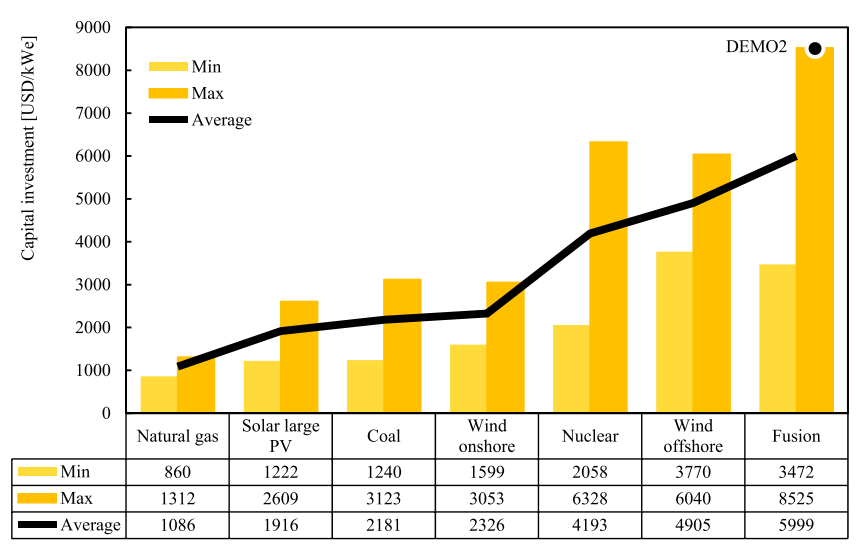There seem to be no recent published cost estimates for current DEMO reactor designs.

### Estimate  by comparison of parts to fission

Crude estimates for the relative costs of fusion and fission can be obtained by comparing the scale of material and the different systems needed for the reactors. The figure below compares the scale of a fusion reactor and a fission reactor with similar, GW, electrical power output.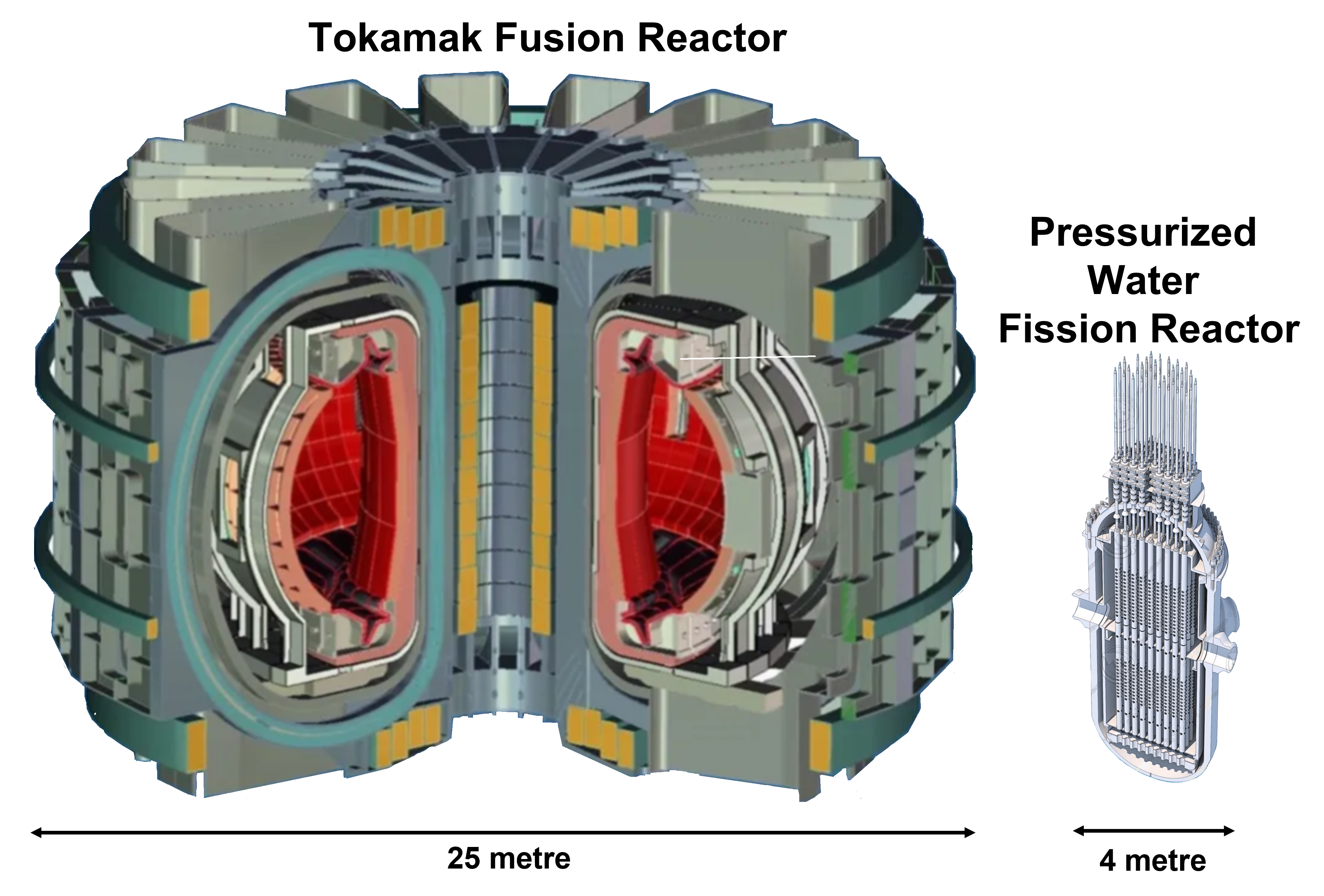Fission reactors are very much smaller than Tokamaks. The former are of the order of 100 m3 while a Tokamak, with similar power output, would be of the order of 10000 m3. This leads to a crude estimate that material costs for a fusion reactor would be about a factor 100 more than fusion. This number does not allow any estimate of manufacturing costs and so can only give the very crude conclusion that it is certain the cost fusion is a lot more than fission.

A slightly less crude estimate, can be based on the breakdown of projected costs for a fusion power plant from the PROCESS reactor design code, given in Nuclear fusion: What of the future? by Richard Kembleton. This breakdown is in the plot below.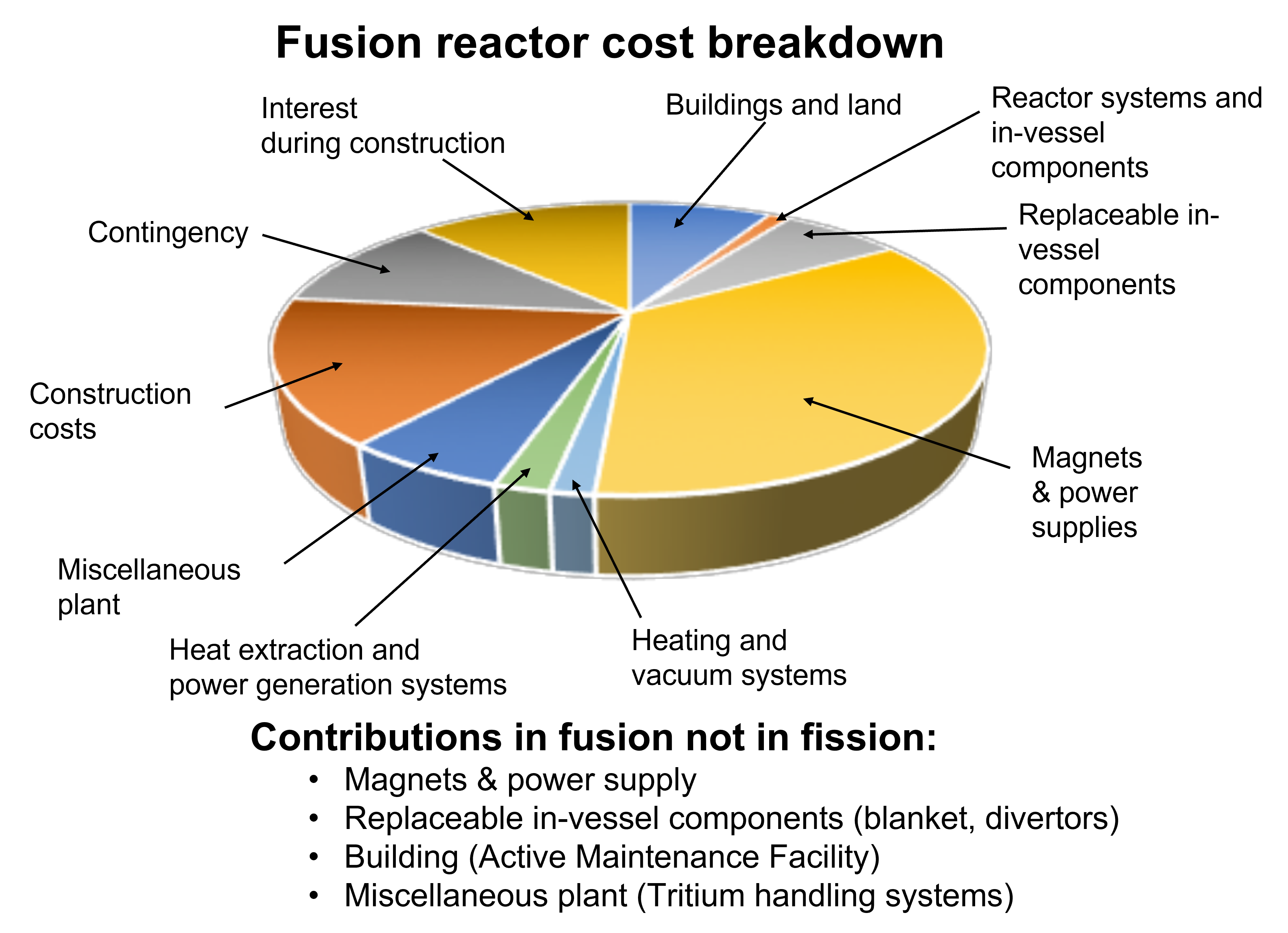Taking into account only component costs (i.e. ignoring construction, contingency and interest), an estimate of the relative cost, fusion to fission, can be made by eliminating items which do not appear in fission. Some items, as indicated above, are dropped completely and for "Building" and "Miscellaneous Plant", a guess of 50% is taken. The items "Heat extraction and power generation systems" as well as "Reactor systems and in-vessel components", are likely similar and so are included in the estimate unchanged. With this, fusion comes out 4.4 times more expensive, for capital investment costs, than fission.

### Cost of operations

Even more difficult to calculate are operational costs. Again an attempt at an estimate can be made by comparing to fission. Fuel costs for fusion for start-up tritium will be astronomical because of the near non-existence of supplies and refining lithium-6 will also be costly. The multiple auxiliary systems of a tokamak such as cryogenics, independent cooling systems; tritium purification plant and frequent replacements, mean high manpower costs and maintenance material costs. The factor relative to fission for all of this will be at least a factor two.

### Electricity production capacity factors

For any electricity generation technology, the proportion of time the power plant produces electricity, "Capacity Factor", is vital in the real electricity cost. The plot below gives indicative values, showing that nuclear (fission) has the highest capacity factor of any technology, in this example >90%.

Capacity factors for different electricity generation technologies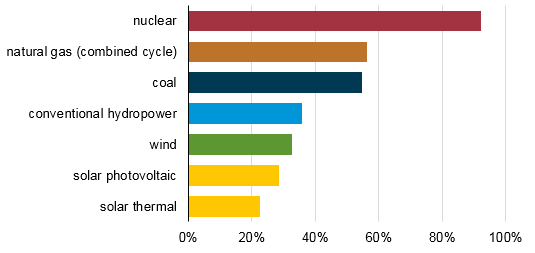For nuclear fusion the capacity factor will be much lower. In the page, Maintenance, Breakdowns and Availability, estimates of the availability are given, which is equivalent to capacity factor. The best guess estimate of capacity factor for fusion is then 30% or 3 times lower than nuclear fission.

### Total cost of fusion compared to fission

This page has shown the construction cost of a fusion power plant would much greater than fission. The volume-based argument is intended to show any suggestions that fusion costs would be similar to fission costs are ridiculous. The other specific estimates made here, say fusion would be at least a factor 4 greater than fission, the operational cost would be at least a factor 2 more than fission and electricity capacity factor at least a factor 3 less than fission.

All this leads to the final electricity costs of fusion being at least several times that of fission. The range of Reinders to Hirsch of a factor 5 to 10, must be taken as the best estimate.